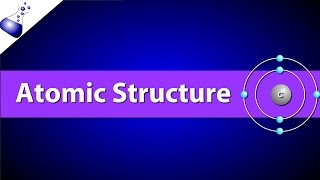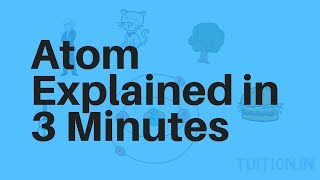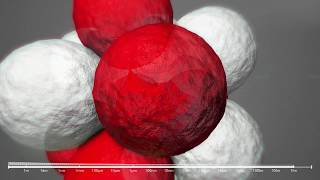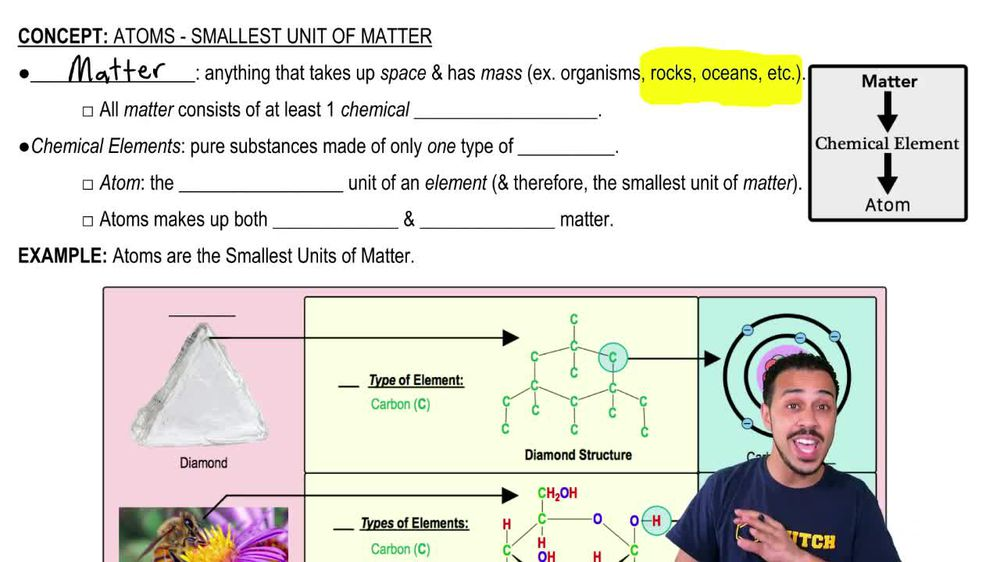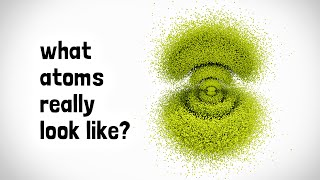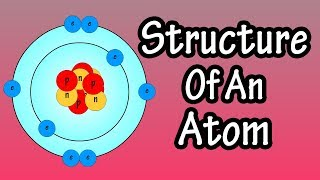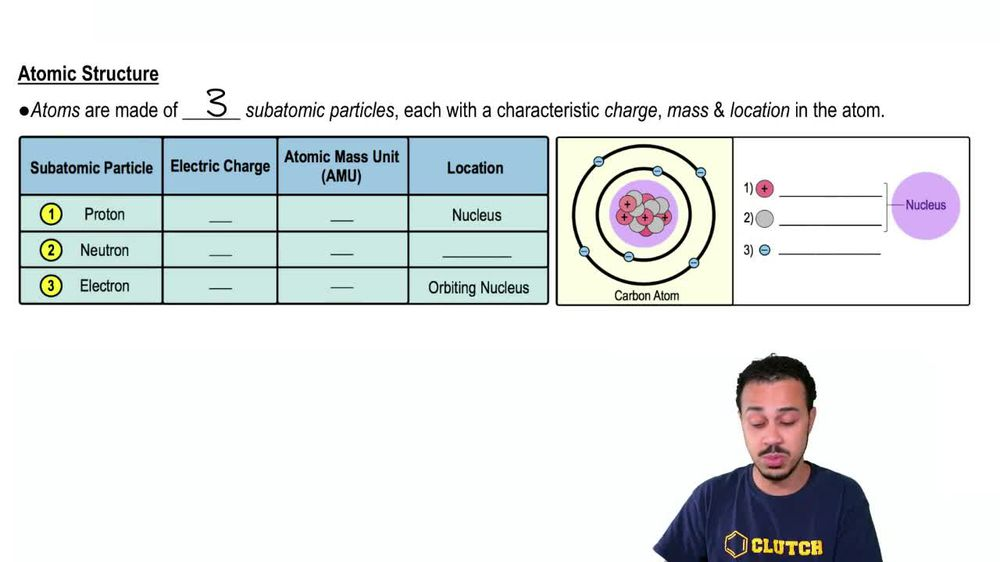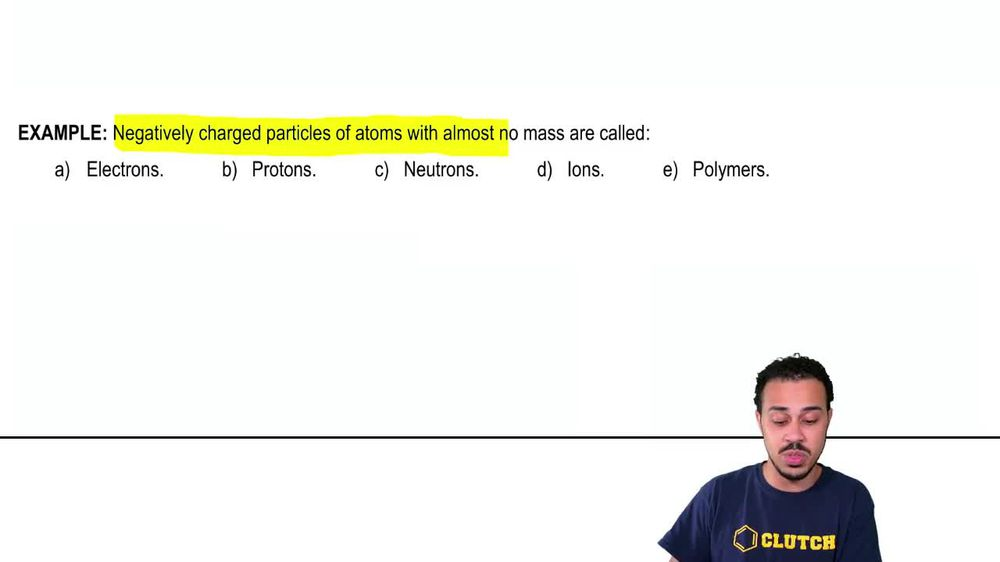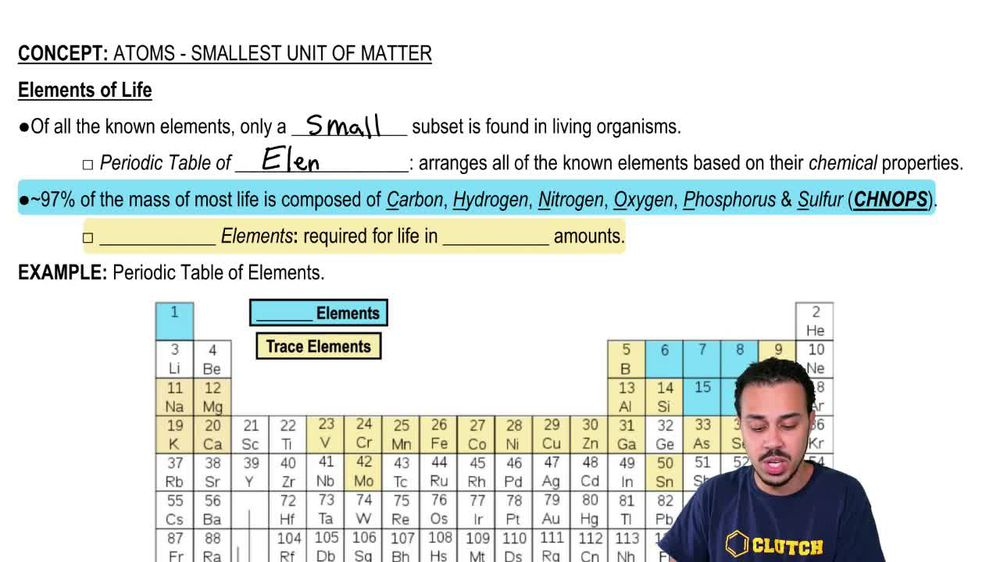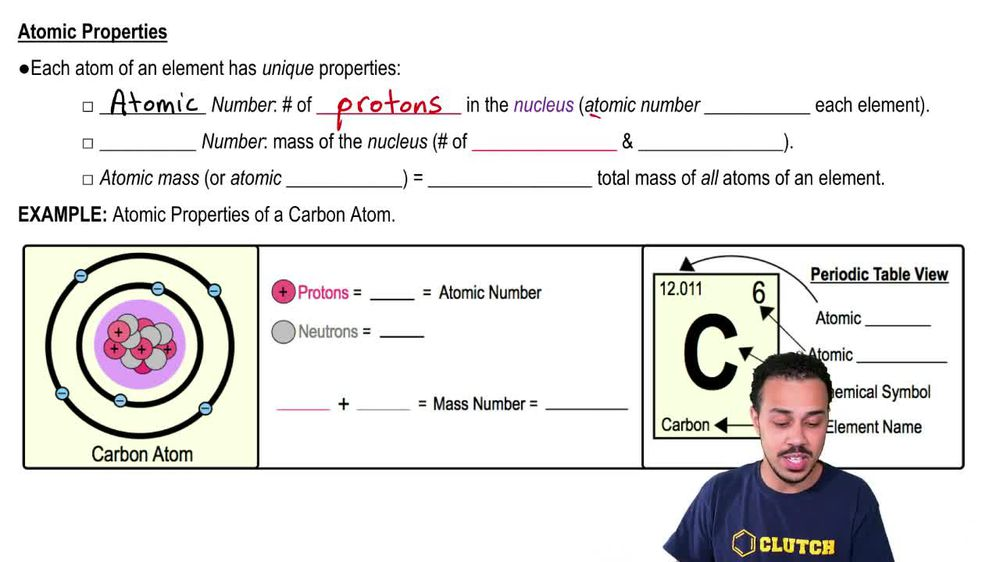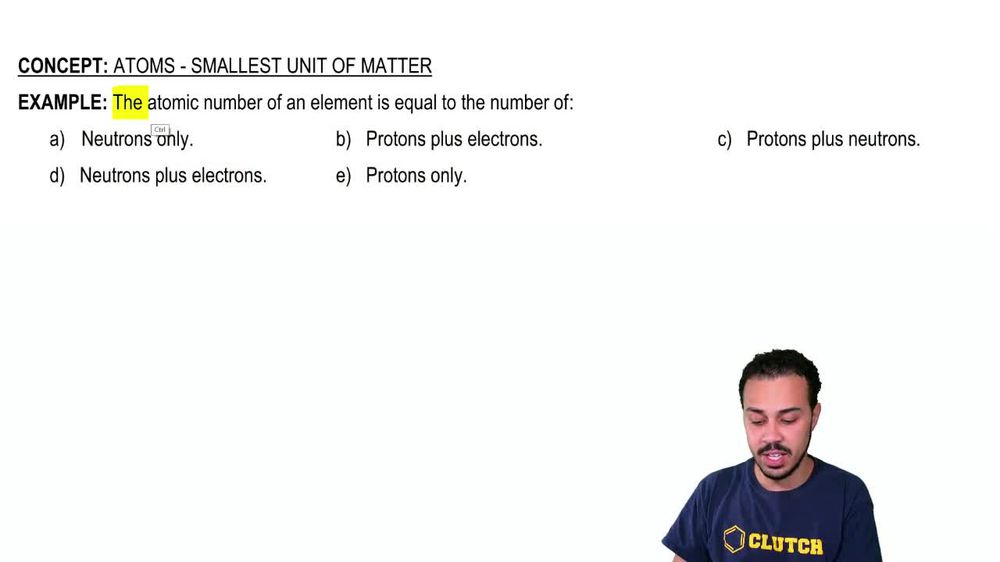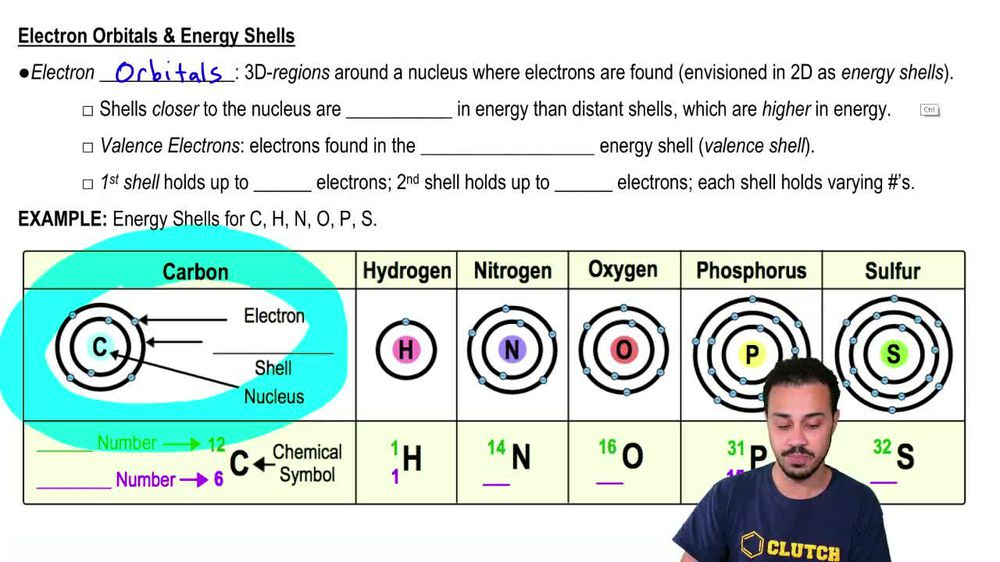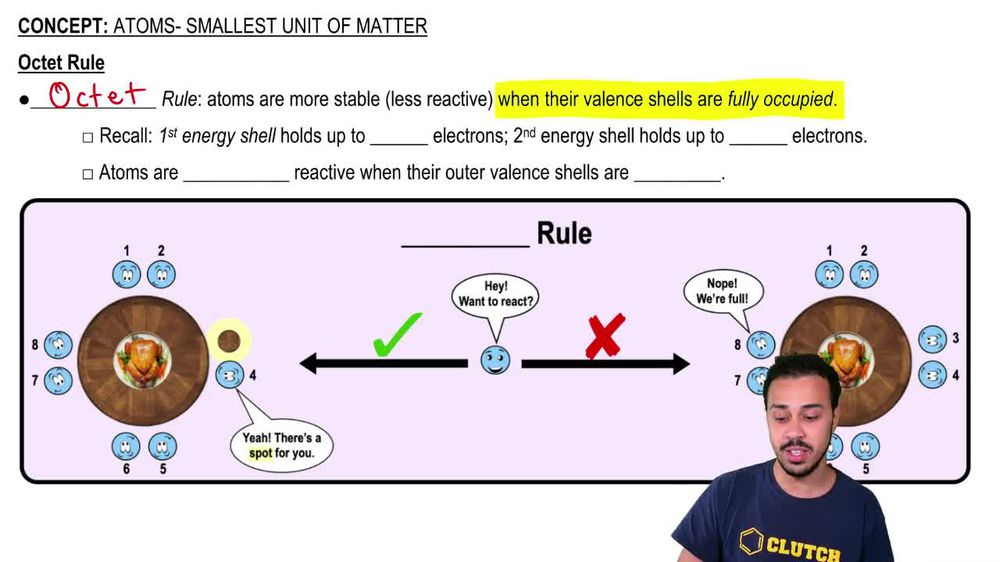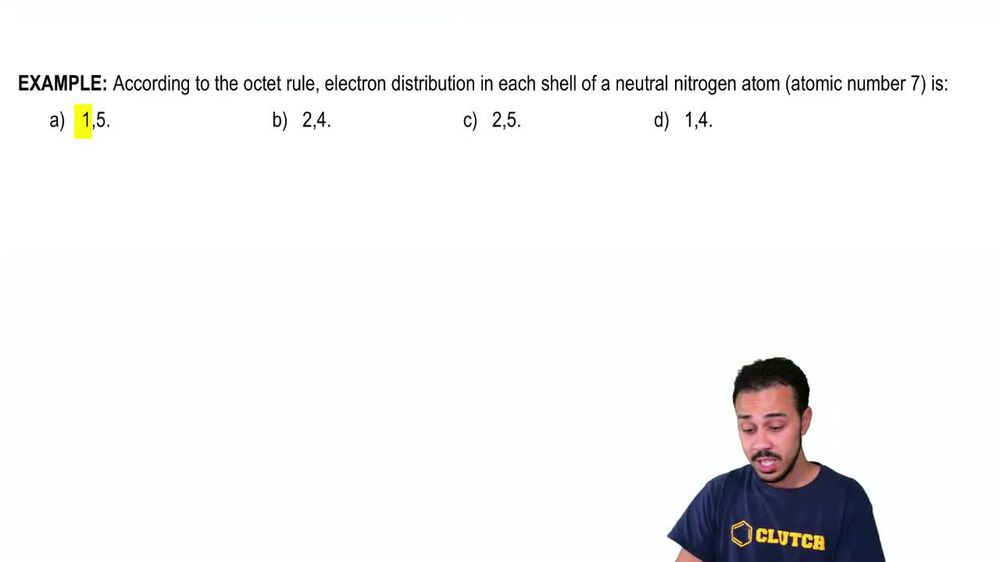Start typing, then use the up and down arrows to select an option from the list.
1. 2. Chemistry2. Atoms- Smallest Unit of Matter# Atoms- Smallest Unit of Matter Example 1

by Jason Amores Sumpter
397 views
10
10
all right. So here we have an example problem that wants us to fill in the sentence here, using one of these five potential answer options down below. And it says that negatively charged particles of Adams with almost no mass are called either electrons, protons, neutrons, ions or polymers. Now, so far, at this point in our course, we have not yet introduced ions or polymers. However, later in our course we definitely will talk about these two terms. But because we have not yet introduced these two terms, we should have been able to figure out that these two are not going to be the correct answer for this problem. So now we're between either electrons, protons or neutrons, which are the three sub atomic particles of a typical Adam recall that the P and protons can remind us that this subatomic particle is positively charged so you can think the P and protons is for the P and positively charged. And so because protons are positively charged, they're not going to be negatively charged, and so we can eliminate, answer, option beat and also recall that neutrons are, as their name implies, new troll and neutral means that it has a net charge of zero, and so it will not be negatively charged. It will have a charge of zero. So once again we can eliminate nutrients. And, of course, this Onley leaves the electrons, which is the correct answer for this example problem. And so electrons are negatively charged and recall that electrons kind of sounds like getting electrocuted and getting electrocuted is something negative. And so that can remind us that electrons are negatively charged. And also electrons have almost no mass. And so we can pretty much say that their mass is about equal to zero. And so we can go ahead and, uh, indicate that a Here is the correct answer to this example problem and that concludes this example. So I'll see you guys in our next video.